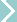Don's HomeReferenceWeights & Measure Conversion factors and equivalents Contact
Common Conversions:
Most of the world has converted to the metric system of measurement [mili-meter, meter, kilometer, liter, gram, kilogram ...], but the U.S. is the only significant holdout. It and a few of it's Caribbean neighbors are still on the English Customary Weights and Measures [inch, foot, yard, mile, gallon, pound, ...]. Size/distance measure in this system is refered to as standard, Inch or SAE (Society of Automotive Engineers)
Distance:
inch = 2.5400 cm [1 cm = 0.39370 in]
mil = 1/1,000 (.001) in
mil = 25.4 micrometer/micron (µm)
1 yd = .91440 meter (m) [1 m = 1.093 613 yd]
mile = 1.609 344 km = 5,280 ft
See size for millimeter (mm),
micrometer/micron (µm), etc.
chain (Gunter, surveyor's, UK) = 66 ft
(18th century US land records)
chain (Ramsden, engineer, US) = 100 ft
AU (Astronomical Unit) = 92.96 M mi
LY light-year = 9.46x1012

Speed:
1 MPH = 1.467 ft/s
1 MPH = 1.609  km/hr = 0.447 m/s
1 km/h = 0.2778 m/s
1 knot = 1.151 MPH

Volume:
liter = 1/1,000 m3 = 61.0 in3 = 0.2642 gal
gallon (US) = 3.785 liter
gallon = 231 in3 = 128 oz
Imperial-gallon (gal-UK) = 1.201 gallon (US)
liquid-quart (qt)= 0.946 liter
quart-dry (qt-dry)= 1.101 liter
oz = 29.57 cm3
4 quart / gallon
2 pints / quart
2 cups / pint
16 oz / pint
8 oz / cup
1 1/2 oz / jigger
2 tablespoon (tbsp) / oz
3 teaspoon (tsp) / tbsp
barrel (petroleum, US) = 42 gallons
(beer, US) = 31 gallons
peck = 8 quarts-dry
bushel = 4 pecks = 35.239 L
Imperial bushel = 36.368 L

Area:
1 acre = 43,560 sq ft
1 sq mi = 640 acres
1 sq km = 247.1 acre
1 hectare = 2.471 acre
See bar measurements in drinks

Flow:
1 gpm = 0.134 ft3/min (cu. ft/min.)
Weight and Mass:
1 pound (lb) = .4536 kg [1 kg=2.205 lbs]
1 short ton = 2,000 lbs
(actually 2000.00000014 lbs.)
1 long ton = 2,240 lbs
1 metric ton = 1,000 kg = 2,205 lbs
1 gallon of water = 8.337 lbs

Force:
1 newton (N) = 0.2248 pound-force
1 kilonewton (kN) = 224.8 lb

Energy - Work:
1 joule = 1 Newton meter (Nm)= 0.7376 ft lb
1 foot pound = 1.356 joule
1 calorie (kcal*) [I.T.] = 4.187 joule
1 calorie (kcal) [I.T.] = 0.003 968 Btu
1 Btu = 252.0 calorie [I.T.]

Power:
1 horsepower = 1,980,000 foot pound-force/hour
1 watt = 860 calories/hour

Pressure:
1 lb/in2 (PSI) = 6.89 kilopascal (kPa)
1 PSI = 2.31 feet head of water
1 PSI = 2.04 in mercury (inHg)
1 N/mm2 = 145.03 PSI
1 Barr = 15 PSI
1 Atmosphere = 2,116 lb/ft2 = 14.70 lb/in2
1 in mercury = 70.7 lb/ft2 = 0.491 lb/in2
1 lb/ft2 = 4.882 kg/mSUP>2

Angular:
1 degree (°) = π/180 (.01745) radians
1 minute (') = 1/60 degree
1 sec (") = 1/60 minute
1 mil=angle whose tangent is 1/1000=.0573°
(i.e. 1 mil at 1 km is 1 m)
1 point = 11.25° [ 8 points = 90°]

Temperature:
Fahrenheight (F) = 1.8*C + 32 (1.8 = 9/5)
Celcius (C) = (F-32) / 1.8
Kelvin (K) = C + 273
 °F 0 32 50 70 90 110 212 °C -18 0 10 21 32 43 100

Illuminance: (degree to which something is illuminated)

1 Footcandle = 1 Lumen (lm)/sq ft
1 Lumen/sq ft = 10.76 LUX (lx) (lumens/sq m)
(energy at light source)
1 candlepower (CANDELA) (cd) = 12.57 lumens (lm)
Surface area of a sphere with radius of 1 ft. is 12.57 sq. ft.
1 watt = 683 lumens (@ 555nm [green]) See Light Brightness Note: Candlepower has been incorrectly used by flashlight manufactures to exaggerate their power.
* kcal - kilocalorie = Amount of heat requird to raise one kilogram of water by one degree Celsius.
The small calorie (gram calorie) is 1/1000 kcal.
Sometimes Calorie (capital C) is used to distinguish kcal from calorie.
When used in the context of food energy the term Calorie generally refers to the kilogram calorie.

Reference Standard:
A kilogram was the mass of water filling a cube that is one-tenth of a meter on each side, or one liter of volume, and a meter was one ten-millionth of the distance from the North Pole to the Equator, along the path passing through Paris (since it was the French Academy of Sciences that defined the meter).

In 1960, scientists defined the meter in terms of the wavelength of a specific orange light emitted by krypton atoms. In 1983, they redefined the speed of light to be exactly 299,792,458 meters per second, so a meter is now just the distance that light travels in a vacuum in 1/299,792,458th of a second.

A platinum-iridium cylinder about the size of a plum LOCKED in a vault in Paris was established as the official definition of the kilogram, in 1889.

In 2005 Scientists at the National Institute of Standards and Technology in Gaithersburg, Md., announced significant progress toward supplanting this cylinder.
It's a two-story-tall contraption that measures the power needed to generate an electromagnetic force that balances the gravitational pull on a kilogram of mass.

See:
Onlineconversion.com
Size for very large and small unit conversions. (Light-year, Astronomical
World Factbook at U. Missouri
KnowledgeDoor.com
Measurement under technology for International System of Units (SI) and notation.
Units of measure, conversion table, converters at allmeasures.com
Metric & English Conversion
Measurement Conversion
Conversion Factors and Formulas Online Conversion:
metric-conversions.org
Institute of Chemistry, FU Berlin
Measurement
Common Weights & Measures

last updated 3 Sep 2007